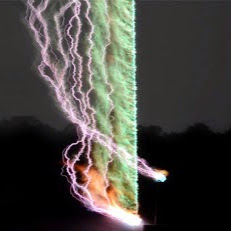# Proof of $(A+B) \times (A-B) = -2(A X B)$

7,332

Using the distributive law, $$(A+B)\times(A-B)=A\times A-A\times B+B\times A-B\times B$$ $$A\times A=B\times B=0$$ $$(A+B)\times(A-B)=0-A\times B+B\times A-0$$ $$B\times A=-A\times B$$ $$(A+B)\times(A-B)=-A\times B-A\times B=-2(A\times B)$$

Share:
7,332Author by

### Shrish Shankar

Hi

Updated on November 14, 2022

•Proof of $(A+B) \times (A-B) = -2(A \times B)$, where 'A' and 'B' are vectors
•